Electron. J. Diff. Equ., Vol. 2011 (2011), No. 125, pp. 1-14.

### Kwong-Wong-type integral equation on time scales Baoguo Jia

Abstract:
Consider the second-order nonlinear dynamic equation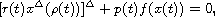where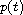is the backward jump operator. We obtain a Kwong-Wong-type integral equation, that is: If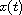is a nonoscillatory solution of the above equation on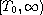, then the integral equation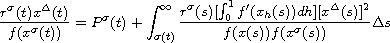is satisfied for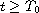, where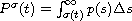, and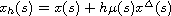. As an application, we show that the superlinear dynamic equationis oscillatory, under certain conditions.

Submitted July 21, 2011. Published September 29, 2011.
Math Subject Classifications: 34K11, 39A10, 39A99.
Key Words: Nonlinear dynamic equation; integral equation; nonoscillatory solution.

Show me the PDF file (263 KB), TEX file, and other files for this article.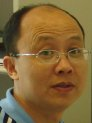Baoguo Jia School of Mathematics and Computer Science Zhongshan University, Guangzhou, 510275, China email: mcsjbg@mail.sysu.edu.cn